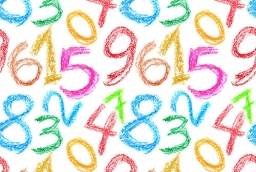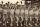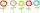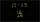# Mixing operations with numbers

Subtract twice the number -23.6 from the difference of the numbers -130 and -40.2.

x =  -42.6

### Step-by-step explanation:Did you find an error or inaccuracy? Feel free to write us. Thank you!## Related math problems and questions:

• I think numberI think number.When I add 841 to it and subtract 157, I get a number that is 22 greater than 996. What number I thinking?
• Area 4gonCalculate the area of 4-gon, two, and the two sides are equal and parallel with lengths 11, 5, 11, and 5. Inner angles are 45°, 135°,45°, 135°.
• AthletesAthletes standing in rows of eight. Ivan estimated that athletes are more than 120. Peter claimed that there are fewer than 130. Both are right. How many are athletes?
• The rawThe raw data presented here are the scores (out of 100 marks) of a market survey regarding the acceptability of new product launched by a company for random sample of 50 respondents: 40 45 41 45 45 30 30 8 48 25 26 9 23 24 26 29 8 40 41 42 39 35 18 25 35
• Unknown xIf we add to unknown number 21, then divide by 6 and then subtract 51, we get back an unknown number. What is this unknown number?
• How manyHow many integers are greater than 547/3 and less than 931/4?
• RegroupingSubtract mixed number with regrouping: 11 17/20- 6 19/20
• RemaindersIt is given a set of numbers { 170; 244; 299; 333; 351; 391; 423; 644 }. Divide this numbers by number 66 and determine set of remainders. As result write sum of this remainders.
• Fractions and mixed numerals(a) Convert the following mixed numbers to improper fractions. i. 3 5/8 ii. 7 7/6 (b) Convert the following improper fraction to mixed number. i. 13/4 ii. 78/5 (c) Simplify these fractions to their lowest terms. i. 36/42 ii. 27/45 2. evaluate following ex
• Divisible by 5How many three-digit odd numbers divisible by 5, which are in place ten's number 3?
• MistakeNicol mistake when calculate in school. Instead of add number 20 subtract it. What is the difference between the result and the right result?
• Unknown number4/5 of a number is 276. what is 2/3 of the same number?
• Reminder and quotientThere are given numbers A = 135, B = 315. Find the smallest natural number R greater than 1 so that the proportions R:A, R:B are with the remainder 1.
• Difference in temperaturesThe temperature in the number line show minus 15 degree celsius and 8 degree celsius. What is the difference between the temperatures?
• ConcertOn a Concert were sold 150 tickets for CZK 360, 235 tickets for 240 CZK and 412 for 180 CZK. How much was the total revenues for tickets?
• FlowersThe flower has six flowers, and each flower has a number. These are the numbers: 20,40,39,28,8,9. What number will be in the middle of the flower so that the numbers come from the flowers when we subtract and add?
• Change the numbers in the ratioChange the numbers 29, 38, and 43 in a 3: 4 ratio.